# Important Questions CBSE Class 9 Maths Chapter 9-Areas of Parallelograms & Triangles

CBSE Class 9 Maths Important questions for chapter 9-Areas of parallelograms and triangles are available here with solutions. These questions have been provided by our subject experts, taking previous years question papers into consideration and as per NCERT book or CBSE syllabus. Students can practice these questions to get good marks in Maths final exam.

Also, they can use these materials as a revision tool for them to have a brief study since there is no time to cover the complete book during the exam. Reach our class 9 Maths important questions only at BYJU’S for free. These CBSE Class 9 Maths Chapter 9 important questions help students to study well and face the exams without any fear.

Also Check:

## Important Questions & Solutions For Class 9 Maths Chapter 9 (Areas of Parallelograms & Triangles)

Q.1. In Figure, ABCD is a parallelogram, AE ⊥ DC and CF ⊥ AD. If AB = 16 cm, AE = 8 cm and CF = 10 cm, find AD.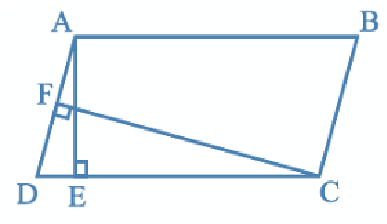Solution:

Given,

AB = CD = 16 cm (Opposite sides of a ||gm are equal)

CF = 10 cm and AE = 8 cm

Now,

Area of parallelogram = Base × Altitude

= CD × AE = AD × CF

⇒ 16 × 8 = AD × 10

Q.2. If E, F, G and H are respectively the mid-points of the sides of a parallelogram ABCD, show that ar (EFGH) = 1/2 ar(ABCD).

Solution: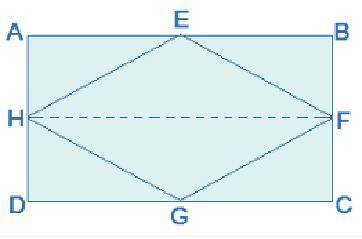Given,

E, F, G and H are the mid-points of the sides of a parallelogram ABCD, respectively.

To Prove: ar (EFGH) = ½ ar(ABCD)

Construction: H and F are joined.

Proof:

AD || BC and AD = BC (Opposite sides of a ||gm)

⇒ ½ AD = ½ BC

Also,

AH || BF and and DH || CF

⇒ AH = BF and DH = CF (H and F are midpoints)

Therefore, ABFH and HFCD are parallelograms.

Now,

As we know, ΔEFH and ||gm ABFH, both lie on the same FH the common base and in-between the same parallel lines AB and HF.

∴ area of EFH = ½ area of ABFH — (i)

And,

area of GHF = ½ area of HFCD — (ii)

area of ΔEFH + area of ΔGHF = ½ area of ABFH + ½ area of HFCD

⇒ ar (EFGH) = ½ ar(ABCD)

Q.3. In Figure, P is a point in the interior of a parallelogram ABCD. Show that

(i) ar(APB) + ar(PCD) = ½ ar(ABCD)

(ii) ar(APD) + ar(PBC) = ar(APB) + ar(PCD)

[Hint : Through P, draw a line parallel to AB.]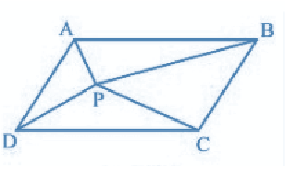Solution: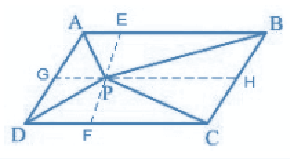(i) A line GH is drawn parallel to AB passing through P.

In a parallelogram,

AB || GH (by construction) — (i)

∴,

AD || BC ⇒ AG || BH — (ii)

From equations (i) and (ii),

ABHG is a parallelogram.

Now,

ΔAPB and ||gm ABHG are lying on the same base AB and in-between the same parallel lines AB and GH.

∴ ar(ΔAPB) = ½ ar(ABHG) — (iii)

also,

ΔPCD and ||gm CDGH are lying on the same base CD and in-between the same parallel lines CD and GH.

∴ ar(ΔPCD) = ½ ar(CDGH) — (iv)

ar(ΔAPB) + ar(ΔPCD) = ½ {ar(ABHG) + ar(CDGH)}

⇒ ar(APB) + ar(PCD) = ½ ar(ABCD)

(ii) A line EF is drawn parallel to AD passing through P.

In the parallelogram,

AD || EF (by construction) — (i)

AB || CD ⇒ AE || DF — (ii)

From equations (i) and (ii),

AEDF is a parallelogram.

Now,

ΔAPD and ||gm AEFD are lying on the same base AD and in-between the same parallel lines AD and EF.

∴ ar(ΔAPD) = ½ ar(AEFD) — (iii)

also,

ΔPBC and ||gm BCFE is lying on the same base BC and in-between the same parallel lines BC and EF.

∴ ar(ΔPBC) = ½ ar(BCFE) — (iv)

ar(ΔAPD) + ar(ΔPBC) = ½ {ar(AEFD) + ar(BCFE)}

⇒ ar(APD) + ar(PBC) = ar(APB) + ar(PCD)

Q.4. In a triangle ABC, E is the mid-point of median AD. Show that ar(BED) = 1/4 ar(ABC).

Solution: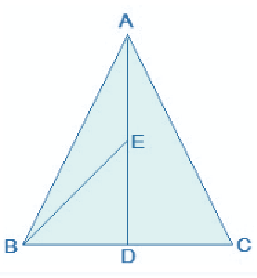ar(BED) = ½ × BD × DE

Since, E is the mid-point of AD,

AE = DE

Since, AD is the median on side BC of triangle ABC,

BD = DC

DE = ½ AD — (i)

BD = ½ BC — (ii)

From (i) and (ii), we get,

ar(BED) = (1/2) × (½) BC × (1/2)AD

⇒ ar(BED) = (½) × (½) ar(ABC)

⇒ ar(BED) = ¼ ar(ABC)

Q.5. In figure, ABC and ABD are two triangles on the same base AB. If line- segment CD is bisected by AB at O, show that: ar(ABC) = ar(ABD).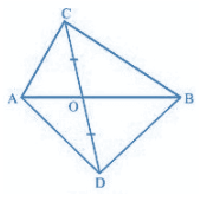Solution:

In ΔABC, AO is the median. (CD is bisected by AB at O)

∴ ar(AOC) = ar(AOD) — (i)

also,

ΔBCD, BO is the median. (CD is bisected by AB at O)

∴ ar(BOC) = ar(BOD) — (ii)

ar(AOC) + ar(BOC) = ar(AOD) + ar(BOD)

⇒ ar(ABC) = ar(ABD)

6. D, E and F are respectively the mid-points of the sides BC, CA and AB of a ΔABC.

Show that

(i) BDEF is a parallelogram.

(ii) ar(DEF) = ¼ ar(ABC)

(iii) ar (BDEF) = ½ ar(ABC)

Solution: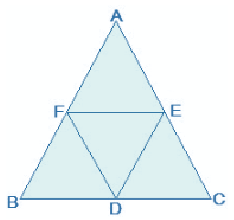(i)In ΔABC,

EF || BC and EF = ½ BC (by midpoint theorem)

also,

BD = ½ BC (D is the midpoint)

So, BD = EF

also,

BF and DE are parallel and equal to each other.

Therefore, the pair opposite sides are equal in length and parallel to each other.

∴ BDEF is a parallelogram.

(ii) Proceeding from the result of (i),

BDEF, DCEF, AFDE are parallelograms.

Diagonal of a parallelogram divides it into two triangles of equal area.

∴ ar(ΔBFD) = ar(ΔDEF) (For ||gm BDEF) — (i)

also,

ar(ΔAFE) = ar(ΔDEF) (For ||gm DCEF) — (ii)

ar(ΔCDE) = ar(ΔDEF) (For ||gm AFDE) — (iii)

From (i), (ii) and (iii)

ar(ΔBFD) = ar(ΔAFE) = ar(ΔCDE) = ar(ΔDEF)

⇒ ar(ΔBFD) + ar(ΔAFE) + ar(ΔCDE) + ar(ΔDEF) = ar(ΔABC)

⇒ 4 ar(ΔDEF) = ar(ΔABC)

⇒ ar(DEF) = ¼ ar(ABC)

(iii)Area (||gm BDEF) = ar(ΔDEF) + ar(ΔBDE)

⇒ ar(BDEF) = ar(ΔDEF) + ar(ΔDEF)

⇒ ar(BDEF) = 2× ar(ΔDEF)

⇒ ar(BDEF) = 2× ¼ ar(ΔABC)

⇒ ar(BDEF) = (1/2)ar(ΔABC)

Q. 7. In figure, diagonals AC and BD of quadrilateral ABCD intersect at O such that OB = OD.

If AB = CD, then show that:

ar (DOC) = ar (AOB)

ar (DCB) = ar (ACB)

DA || CB or ABCD is a parallelogram.

[Hint: From D and B, draw perpendiculars to AC.]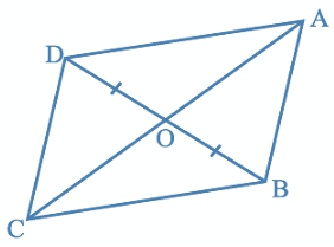Solution: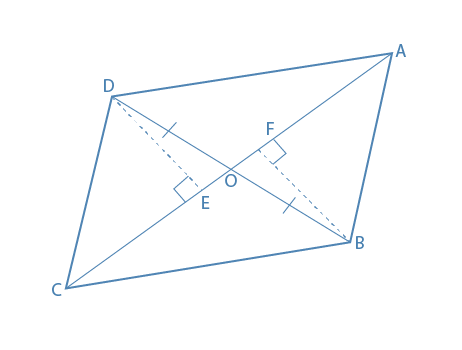Given: OB = OD and AB = CD

Construction: DE ⊥ AC and BF ⊥ AC are drawn.

Proof:

(i) In ΔDOE and ΔBOF,

∠DEO = ∠BFO (Perpendiculars)

∠DOE = ∠BOF (Vertically opposite angles)

OD = OB (Given)

∴, ΔDOE ≅ ΔBOF (by AAS congruence criterion)

∴, DE = BF (By CPCT) — (1)

also, ar(ΔDOE) = ar(ΔBOF) (Congruent triangles) — (2)

Now,

In ΔDEC and ΔBFA,

∠DEC = ∠BFA (Perpendiculars)

CD = AB (Given)

DE = BF (From eq.1)

∴, ΔDEC ≅ ΔBFA (by RHS congruence criterion)

∴, ar(ΔDEC) = ar(ΔBFA) (Congruent triangles) — (3)

ar(ΔDOE) + ar(ΔDEC) = ar(ΔBOF) + ar(ΔBFA)

⇒ ar (DOC) = ar (AOB)

(ii) ar(ΔDOC) = ar(ΔAOB)

Adding ar(ΔOCB) in LHS and RHS, we get,

⇒ ar(ΔDOC)+ar(ΔOCB)=ar(ΔAOB)+ar(ΔOCB)

⇒ ar(ΔDCB) = ar(ΔACB)

(iii) When two triangles have the same base and equal areas, the triangles will be in between the same parallel lines

ar(ΔDCB) = ar(ΔACB)

DA || BC — (4)

For quadrilateral ABCD, one pair of opposite sides are equal (AB = CD) and other pair of opposite sides are parallel.

∴, ABCD is a parallelogram.

Q. 8. XY is a line parallel to side BC of a triangle ABC. If BE || AC and CF || AB meet XY at E and F respectively, show that

ar(ΔABE) = ar(ΔACF)

Solution: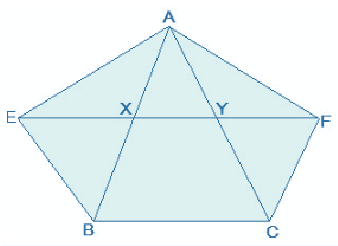Given: XY || BC, BE || AC and CF || AB

To show: ar(ΔABE) = ar(ΔACF)

Proof:

BCYE is a ||gm as ΔABE and ||gm BCYE are on the same base BE and between the same parallel lines BE and AC.

∴, ar(ABE) = ½ ar(BCYE) … (1)

Now,

CF || AB and XY || BC

⇒ CF || AB and XF || BC

⇒ BCFX is a parallelogram

As ΔACF and ||gm BCFX are on the same base CF and in-between the same parallel AB and FC.

∴, ar (ΔACF)= ½ ar (BCFX) … (2)

But,

||gm BCFX and ||gm BCYE are on the same base BC and between the same parallels BC and EF.

∴, ar (BCFX) = ar(BCYE) … (3)

From (1) , (2) and (3) , we get

ar (ΔABE) = ar(ΔACF)

⇒ ar(BEYC) = ar(BXFC)

As the parallelograms are on the same base BC and in-between the same parallels EF and BC…..(4)

Also,

△AEB and ||gm BEYC is on the same base BE and in-between the same parallels BE and AC.

⇒ ar(△AEB) = ½ ar(BEYC) ……(5)

Similarly,

△ACF and ||gm BXFC on the same base CF and between the same parallels CF and AB.

⇒ ar(△ ACF) = ½ ar(BXFC) ……..(6)

From (4), (5) and (6),

ar(△AEB) = ar(△ACF)

Q.9. Parallelogram ABCD and rectangle ABEF are on the same base AB and have equal areas. Show that the perimeter of the parallelogram is greater than that of the rectangle.

Solution: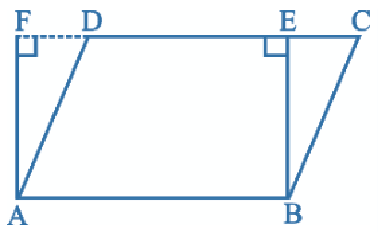Given,

||gm ABCD and a rectangle ABEF have the same base AB and equal areas.

To prove,

The perimeter of ||gm ABCD is greater than the perimeter of rectangle ABEF.

Proof,

As we know, the opposite sides of a||gm and rectangle are equal.

AB = DC [As ABCD is a ||gm]

and, AB = EF [As ABEF is a rectangle]

DC = EF … (i)

Adding AB on both sides, we get,

⇒ AB + DC = AB + EF … (ii)

As we know, the perpendicular segment is the shortest of all the segments that can be drawn to a given line from a point not lying on it.

BE < BC and AF < AD

⇒ BC > BE and AD > AF

⇒ BC + AD > BE + AF … (iii)

Adding (ii) and (iii), we get

AB + DC + BC + AD > AB + EF + BE + AF

⇒ AB + BC + CD + DA > AB + BE + EF + FA

⇒ perimeter of ||gm ABCD > perimeter of rectangle ABEF.

The perimeter of the parallelogram is greater than that of the rectangle.

Q.10. Diagonals AC and BD of a quadrilateral ABCD intersect each other at E. Show that

ar(ΔAED) × ar(ΔBEC) = ar (ΔABE) × ar (ΔCDE).

[Hint: From A and C, draw perpendiculars to BD.]

Solution:

Given: The diagonal AC and BD of the quadrilateral ABCD, intersect each other at point E.

Construction:

From A, draw AM perpendicular to BD

From C, draw CN perpendicular to BD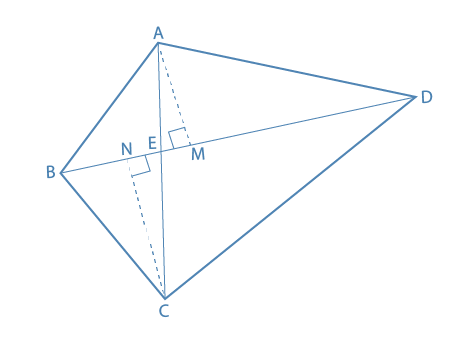To Prove: ar(ΔAED) ar(ΔBEC) = ar (ΔABE) ar (ΔCDE)

Proof:

ar(ΔABE) = ½ ×BE×AM………….. (i)

ar(ΔAED) = ½ ×DE×AM………….. (ii)

Dividing eq. (ii) by (i) , we get,

ar(ΔAED)/ar(ΔABE) = [1/2×DE×AM]/ [1/2×BE×AM]

= DE/BE ………..(iii)

Similarly,

ar(ΔCDE)/ar(ΔBEC) = DE/BE …….(iv)

From eq. (iii) and (iv), we get;

ar(ΔAED)/ar(ΔABE) = ar(ΔCDE)/ar(ΔBEC)​

Therefore, ar(ΔAED) × ar(ΔBEC) = ar (ΔABE) × ar (ΔCDE)

### Extra Questions for Class 9 Maths Chapter 9

1. P and Q are any two points lying on the sides DC and AD respectively of a parallelogram ABCD. Show that ar(APB) = ar(BQC).
2. PQRS and ABRS are parallelograms and X is any point on side BR. Show that:
• ar(PQRS) = ar(ABRS)
• ar (AXS) = ½ ar(PQRS)
3. A farmer was having a field in the form of a parallelogram PQRS. She took any point A on RS and joined it to points P and Q. In how many parts the field is divided? What are the shapes of these parts? The farmer wants to sow wheat and pulses in equal portions of the field separately. How should she do it?
4. In Figure, E is any point on the median AD of a ΔABC. Show that ar (ABE) = ar(ACE).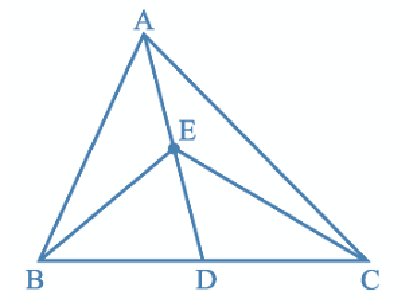5. Show that the diagonals of a parallelogram divide it into four triangles of equal area.
6. D and E are points on sides AB and AC respectively of ΔABC such that ar(DBC) = ar(EBC). Prove that DE || BC.
7. The side AB of a parallelogram ABCD is produced to any point P. A line through A and parallel to CP meets CB produced at Q and then parallelogram PBQR is completed (see in the figure). Show that:
• ar(ABCD) = ar(PBQR).
• [Hint: Join AC and PQ. Now compare ar(ACQ) and ar(APQ).]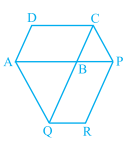8. Diagonals AC and BD of a trapezium ABCD with AB || DC intersect each other at O. Prove that ar (AOD) = ar (BOC).